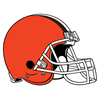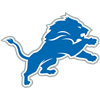2021 NFL Offensive Player of the Year Odds
+175
1.75 to 1Cooper Kupp
22.7% implied probability

+275
2.75 to 1Jonathan Taylor
16.7% implied probability

+1000
10 to 1Deebo Samuel
5.7% implied probability

+2000
20 to 1Austin Ekeler
3.0% implied probability

+2000
20 to 1James Conner
3.0% implied probability

+2000
20 to 1Davante Adams
3.0% implied probability

+2000
20 to 1Josh Allen
3.0% implied probability

+2500
25 to 1Justin Herbert
2.4% implied probability

+3500
35 to 1Kyler Murray
1.7% implied probability

+3500
35 to 1Lamar Jackson
1.7% implied probability

+3500
35 to 1Dak Prescott
1.7% implied probability

+3500
35 to 1Matthew Stafford
1.7% implied probability

+3500
35 to 1Tom Brady
1.7% implied probability

+4000
40 to 1Justin Jefferson
1.5% implied probability

+4000
40 to 1Mike Evans
1.5% implied probability

+4000
40 to 1Tyreek Hill
1.5% implied probability

+5000
50 to 1Ja'Marr Chase
1.2% implied probability

+5000
50 to 1Aaron Rodgers
1.2% implied probability

+5000
50 to 1Jalen Hurts
1.2% implied probability

+5000
50 to 1Nick Chubb
1.2% implied probability

+5000
50 to 1Dalvin Cook
1.2% implied probability

+6500
65 to 1Joe Burrow
0.9% implied probability

+6500
65 to 1CeeDee Lamb
0.9% implied probability

+6500
65 to 1DK Metcalf
0.9% implied probability

+6500
65 to 1Patrick Mahomes
0.9% implied probability

+6500
65 to 1Aaron Jones
0.9% implied probability

+6500
65 to 1Stefon Diggs
0.9% implied probability

+8000
80 to 1Travis Kelce
0.8% implied probability

+8000
80 to 1Joe Mixon
0.8% implied probability

+8000
80 to 1DeAndre Hopkins
0.8% implied probability

+8000
80 to 1Alvin Kamara
0.8% implied probability

+10000
100 to 1Marquise Brown
0.6% implied probability

+10000
100 to 1Ryan Tannehill
0.6% implied probability

+10000
100 to 1DJ Moore
0.6% implied probability

+10000
100 to 1Mike Williams
0.6% implied probability

+10000
100 to 1Kirk Cousins
0.6% implied probability

+10000
100 to 1Keenan Allen
0.6% implied probability

+10000
100 to 1Najee Harris
0.6% implied probability

+10000
100 to 1Mac Jones
0.6% implied probability

+10000
100 to 1Derek Carr
0.6% implied probability

+10000
100 to 1Adam Thielen
0.6% implied probability

+10000
100 to 1Melvin Gordon
0.6% implied probability

+10000
100 to 1Darren Waller
0.6% implied probability

+10000
100 to 1Ezekiel Elliott
0.6% implied probability

+10000
100 to 1D'Andre Swift
0.6% implied probability

+10000
100 to 1Damien Harris
0.6% implied probability

+10000
100 to 1Carson Wentz
0.6% implied probability

+10000
100 to 1Terry McLaurin
0.6% implied probability

+10000
100 to 1A.J. Brown
0.6% implied probability

+10000
100 to 1Leonard Fournette
0.6% implied probability

+10000
100 to 1Kyle Pitts
0.6% implied probability